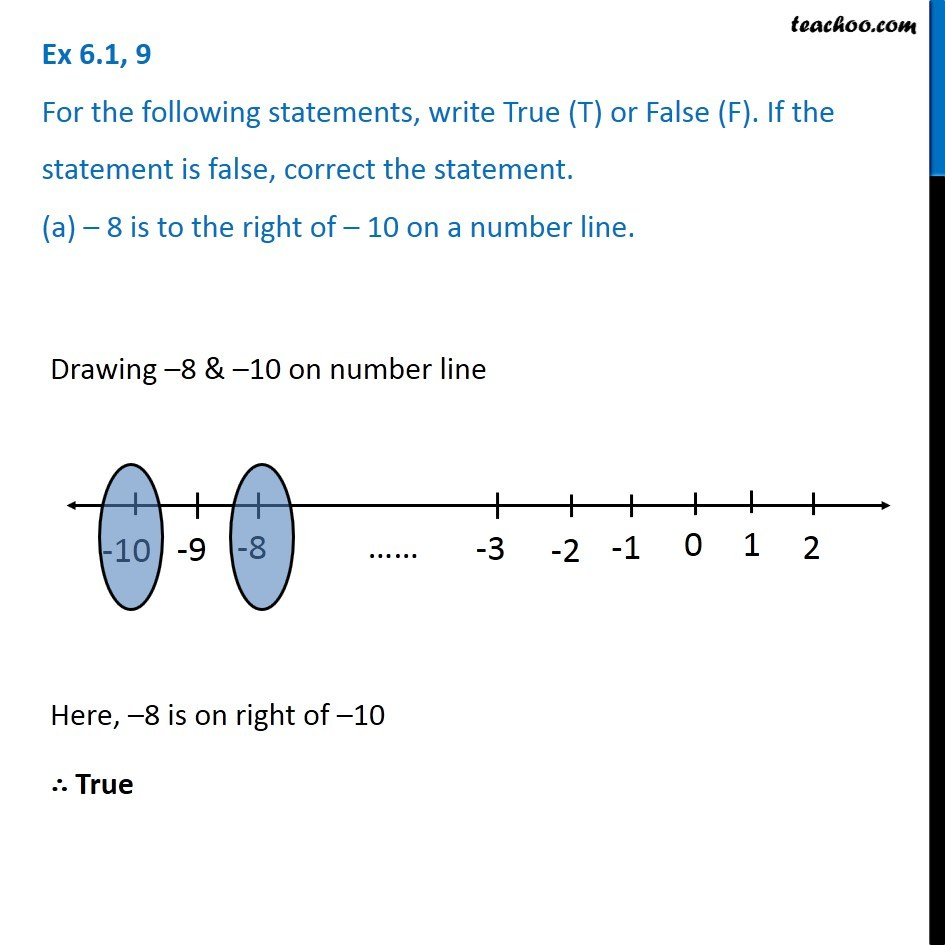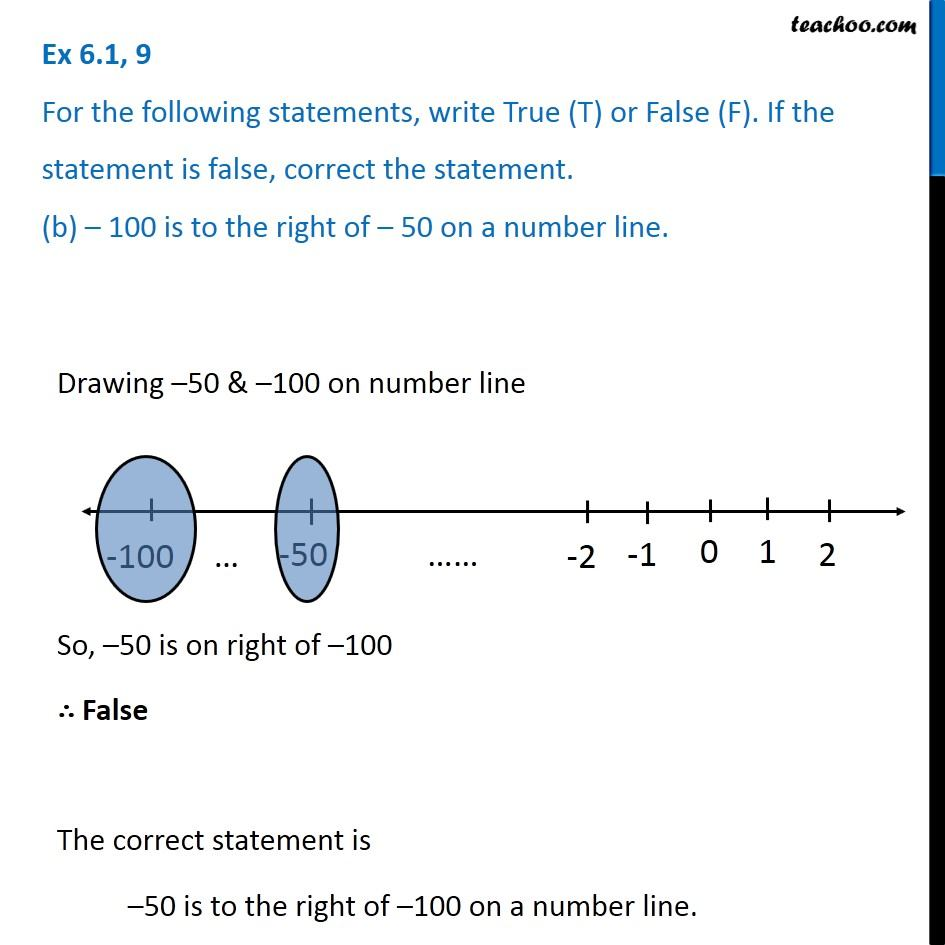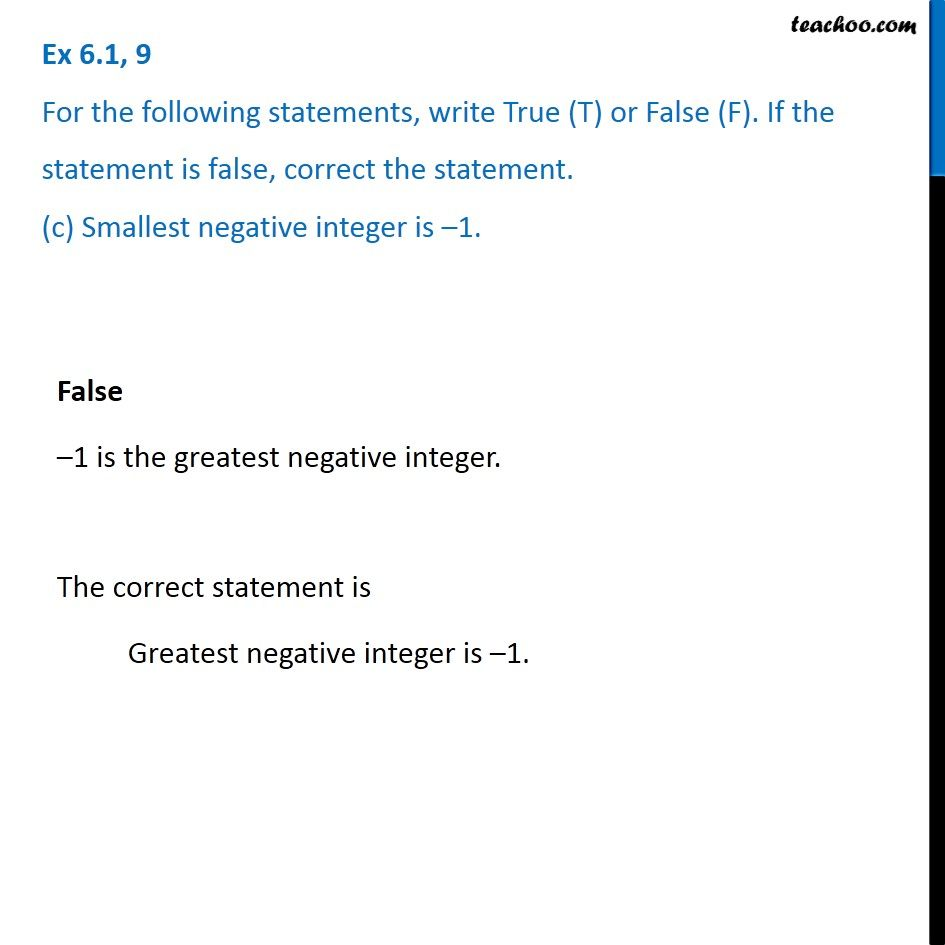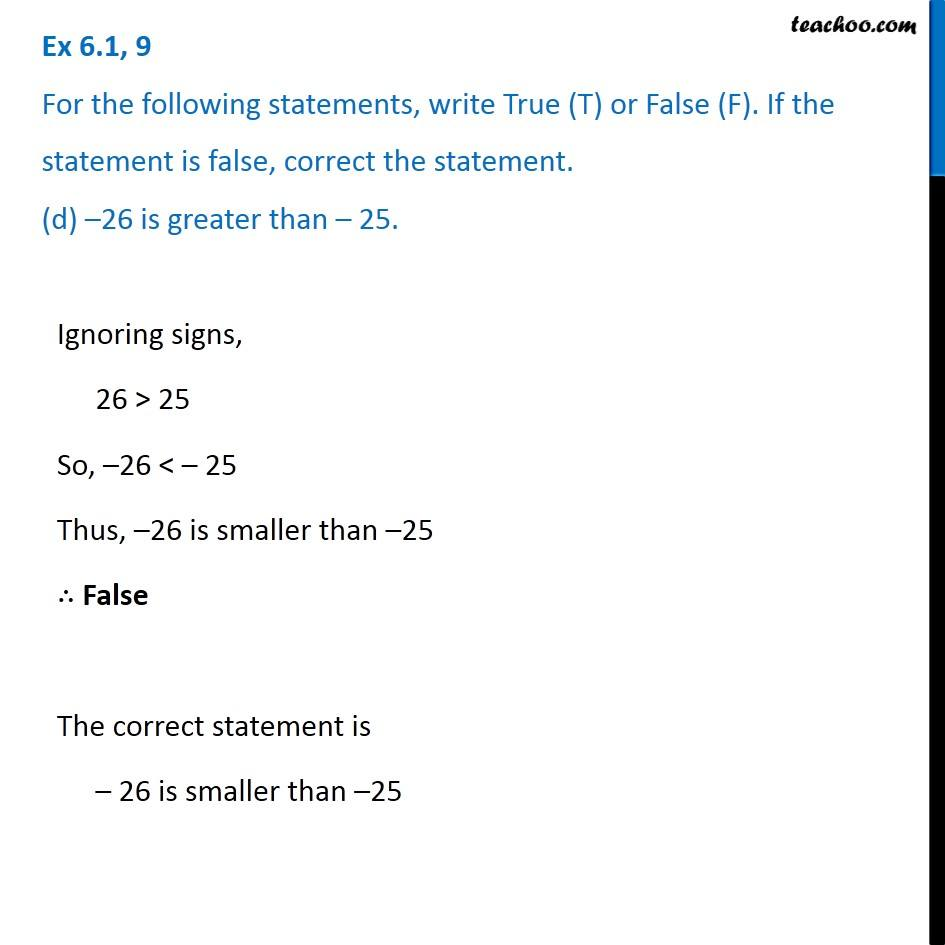Subscribe to our Youtube Channel - https://you.tube/teachoo

1. Chapter 6 Class 6 Integers
2. Concept wise
3. Greater than smaller than in integers

Transcript

Ex 6.1, 9 For the following statements, write True (T) or False (F). If the statement is false, correct the statement. (a) – 8 is to the right of – 10 on a number line. Drawing –8 & –10 on number line Here, –8 is on right of –10 ∴ True Ex 6.1, 9 For the following statements, write True (T) or False (F). If the statement is false, correct the statement. (b) – 100 is to the right of – 50 on a number line. Drawing –50 & –100 on number line So, –50 is on right of –100 ∴ False The correct statement is –50 is to the right of –100 on a number line. Ex 6.1, 9 For the following statements, write True (T) or False (F). If the statement is false, correct the statement. (c) Smallest negative integer is –1. False –1 is the greatest negative integer. The correct statement is Greatest negative integer is –1. Ex 6.1, 9 For the following statements, write True (T) or False (F). If the statement is false, correct the statement. (d) –26 is greater than – 25.Ignoring signs, 26 > 25 So, –26 < – 25 Thus, –26 is smaller than –25 ∴ False The correct statement is – 26 is smaller than –25

Greater than smaller than in integers